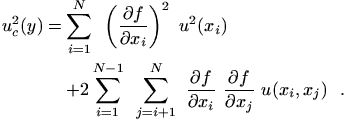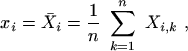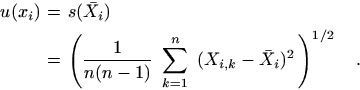An official website of the United States governmentThe .gov means it’s official.
Federal government websites often end in .gov or .mil. Before sharing sensitive information, make sure you’re on a federal government site.The site is secure.
The https:// ensures that you are connecting to the official website and that any information you provide is encrypted and transmitted securely.

# NIST TN 1297: Appendix A. Law of Propagation of Uncertainty

## Share

A.1 In many cases a measurand Y is not measured directly, but is determined from N other quantities X1, X2, ... , XN through a functional relation f:

$$Y = f(X_1, ~X_2, ~\ldots, ~X_N) ~ .$$

(A-1)

Included among the quantities Xi are corrections (or correction factors) as described in subsection 5.2, as well as quantities that take into account other sources of variability, such as different observers, instruments, samples, laboratories, and times at which observations are made (e.g., different days). Thus the function f of Eq. (A-1) should express not simply a physical law but a measurement process, and in particular, it should contain all quantities that can contribute a significant uncertainty to the measurement result.

A.2 An estimate of the measurand or output quantity Y, denoted by y, is obtained from Eq. (A-1) using input estimates x1, x2 , ... , xN for the values of the N input quantities X1, X2, ... , XN. Thus the output estimate y, which is the result of the measurement, is given by

$$y = f(x_1, ~x_2, ~\ldots, ~x_N) ~ .$$

(A-2)

A.3 The combined standard uncertainty of the measurement result y, designated by uc(y) and taken to represent the estimated standard deviation of the result, is the positive square root of the estimated variance uc2(y) obtained from(A-3)

Equation (A-3) is based on a first-order Taylor series approximation of Y = f (X1, X2, ... , XN) and is conveniently referred to as the law of propagation of uncertainty. The partial derivatives ∂f/∂xi (often referred to as sensitivity coefficients) are equal to ∂f/∂Xi evaluated at Xi  = xi ; u(xi ) is the standard uncertainty associated with the input estimate xi ; and u(xi , xj ) is the estimated covariance associated with xi and xj .

A.4 As an example of a Type A evaluation, consider an input quantity Xi whose value is estimated from n independent observations Xi,k of Xi obtained under the same conditions of measurement. In this case the input estimate xi is usually the sample mean(A-4)

and the standard uncertainty u(xi ) to be associated with xi is the estimated standard deviation of the mean(A-5)

A.5 As an example of a Type B evaluation, consider an input quantity Xi whose value is estimated from an assumed rectangular probability distribution of lower limit a- and upper limit a+. In this case the input estimate is usually the expectation of the distribution

$$x_i = (a_+ + a_-)/2 ~ ,$$

(A-6)

and the standard uncertainty u(xi ) to be associated with xi is the positive square root of the variance of the distribution

$$u(x_i) = a/\sqrt{3} ~ ,$$

(A-7)

where a = (a+ - a-)/2 (see subsection 4.6).

NOTE -- When xi is obtained from an assumed distribution, the associated variance is appropriately written as u2(Xi ) and the associated standard uncertainty as u(Xi ), but for simplicity, u2(xi) and u(xi ) are used. Similar considerations apply to the symbols uc2(y) and uc(y).

## Contacts

• PML webmaster
Created November 6, 2015, Updated November 25, 2019